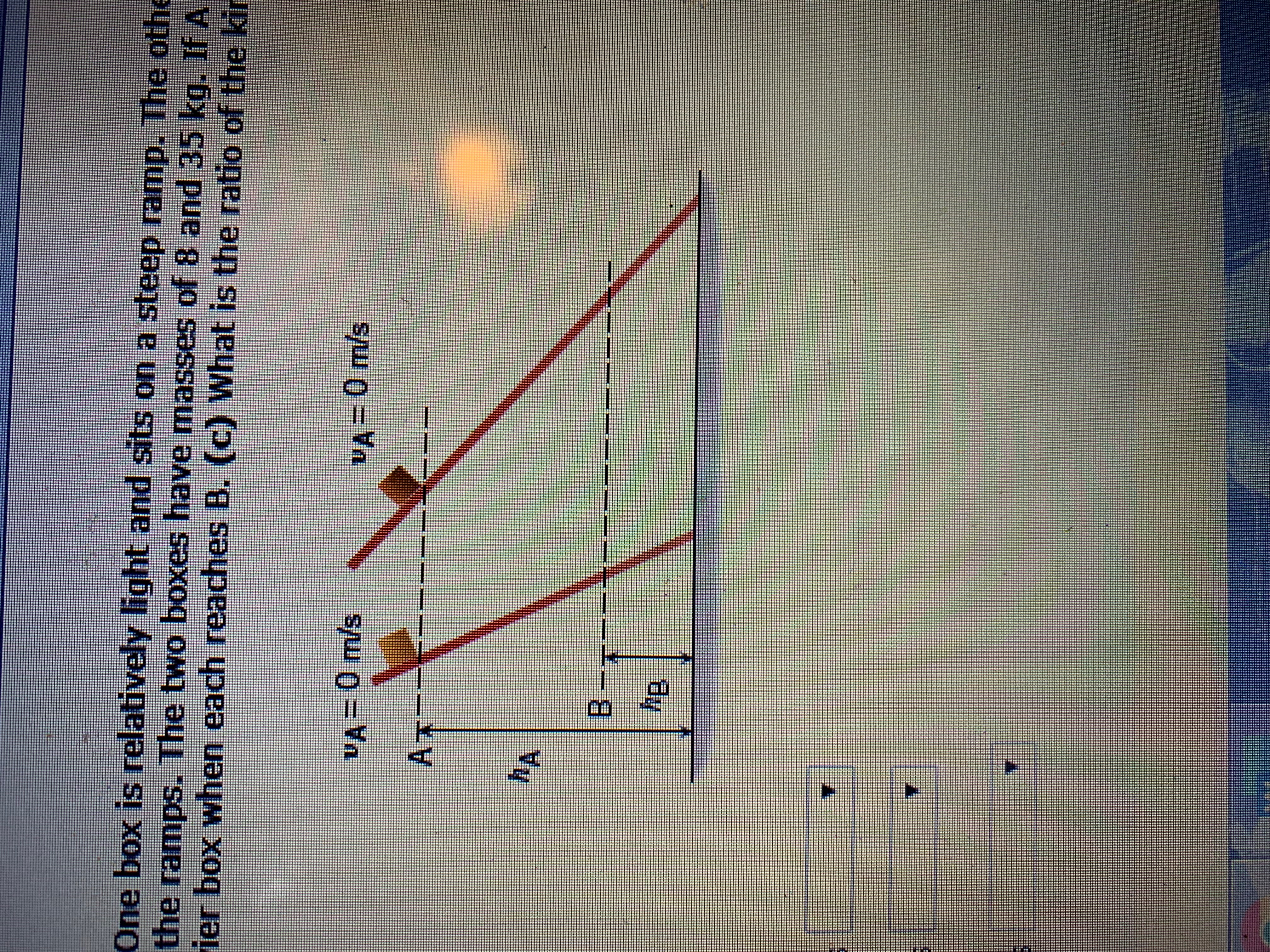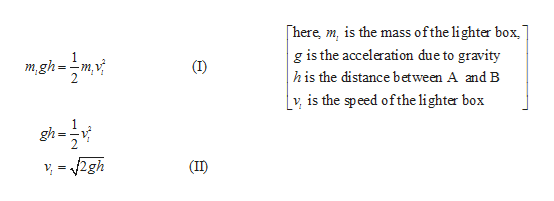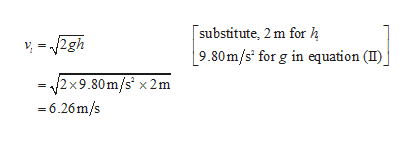One box is relatively light and sits on a steep ramp. The othethe ramps. The two boxes have masses of 8 and 35 kq. If Aier box when each reaches B. (C) What is the ratio of the kirA-0m/sVA= 0 m/sAB -A

Question

The drawing shows two boxes resting on frictionless ramps. One box is relatively light and sits on a steep ramp. The other box is heavier and rests on a ramp that is less steep. The boxes are released from rest at A and allowed to slide down the ramps. The two boxes have masses of 8 and 35 kg. If A and B are 3.0 and 1.0 m, respectively, above the ground, determine the speed of (a) the lighter box and (b) the heavier box when each reaches B. (c) What is the ratio of the kinetic energy of the heavier box to that of the lighter box at B?help_outlineImage TranscriptioncloseOne box is relatively light and sits on a steep ramp. The othe the ramps. The two boxes have masses of 8 and 35 kq. If A ier box when each reaches B. (C) What is the ratio of the kir A-0m/s VA= 0 m/s A B - A fullscreen
Step 1

The mass of the lighter box = 8 kg.

The mass of the heavier box = 35 kg.

The distance between point A and B =3 m-1 m

=2 m.

(a) As the box slides from point A to point B the potential energy changes to kinetic energy. According to conservation of energy the kinetic energy will be equal to potential energy.

Consider equation (I), and obtain an expression for speed of the lighter box.help_outlineImage Transcriptioncloseofthe lighter box here, m, is the mass g is the acceleration due to gravity his the distance between A and B v is the speed ofthe li ghter box m,gh (IID fullscreen
Step 2

Substitute the corresponding values.help_outlineImage Transcriptionclosesubstitute, 2 m for h 2gh 9.80m/s for g in equation (I)j = 2x9.80m/s x 2m 6.26m/s fullscreen
Step 3

(b) Since equation (II) is independent of mass, ...

Want to see the full answer?

See Solution

Want to see this answer and more?

Our solutions are written by experts, many with advanced degrees, and available 24/7

See Solution
Tagged in

Physics